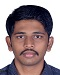# Mathematical Relation for Equilibrium State in Thermodynamics SystemsText Only Version

#### Mathematical Relation for Equilibrium State in Thermodynamics Systems

Arjun M

Chennai, India

Abstract: All basic systems in thermodynamics are governed by all basic laws of thermodynamics that is subjects relating to heat and energy. This work explains in details about mathematical relation which governs basic laws of thermodynamics. The specification goes in detail for zeroth law of thermodynamics. It comes from transitive relation between the thermodynamics systems. This laws title was invented by Arnold Somerfield and Ralph Folpher. Two systems will be in the relation of thermal equilibrium if there exist a wall permeable only to heat and they do not change over time. It tells about thermal equilibrium equivalence relation.

Keywords: Thermal Equilibrium

I.INTRODUCTION

Initially, Maxwell stated that All diathermal walls are equivalent which tells about boundary of the thermodynamic system. Later, Arnold Somerfield and Ralph Folpher summaries the theory of thermal equilibrium and coined the title Zeroth law of Thermodynamics. Later, the law was postulated for heat. This work details about mathematical relation about zeroth law of thermodynamics. It follows the concept of transitive relation. The mathematical proof for 3 or more thermal equilibriums is explained in this work. It includes all boundaries, systems and universe. All expressions with relational statements are explained in this work. The mathematical expression or relation explaining this statement When A is in equilibrium with B, when B is in equilibrium with C and then A is in equilibrium with C.

1. LITERATURE SURVEY

The work by Denis J Evans, Stephen R Williamson and Lomborto Rondoni gives the information that they identify the temperature of a statistical-mechanical system, and forms a cornerstone of thermodynamics. Later works shows that Recently, it has been shown mathematically that a non- isothermal system relaxes to canonical equilibrium conditions, with all components of the system having the same temperature.

The work by Abelardo Ramirez Hernandez infers that method in which zeroth law of thermodynamics is violated. It states that negative specific heat violates the thermodynamics zeroth law. The final equilibrium is such that two phases appear; that is, the subsystems have different magnetizations and internal energies at temperatures which

are equal in both systems, but that can be different from the initial temperature.

In the work titled Zeroth law compatibility of non-additive thermodynamics by T. Biro and P. Van states that zeroth law of thermodynamics is not valid for non-additive Composition materials. From this work it is known that zeroth law of thermodynamics is an axiom and valid to all ordinary thermal equilibriums. It is valid for systems with non additive composition materials contained in thermodynamics.

In the work titled Counting transitive Relation by Gotz Pfeiffer shows the number of possible relations in a transitive relation.

2. MATHEMATICAL RELATION OF ZEROTH LAW OF THERMODYNAMICS

1. It is valid only for thermal equilibrium.

2. It is also applicable under the conditions that materials are of non-additive composition. thermodynamics materials.

3. It is not valid for systems with the conditions with negative specific heat.

Lets first take 3 systems A, B, C under the universe with boundaries.

Transitive relation is applied to these 3 systems.

Lets say a relation R is in the Universe (universal set) U

(A, B) R

(B, C) R

(A, C) R

For all system A, B, C U

Hence, it is proved that zeroth law of thermodynamics is valid only for systems belongs to the universe. If A is equilibrium with B, B is in equilibrium with C, then A is in equilibrium with C. It is valid only if the systems are of thermal equilibrium, non- negative specific heats and additive composition.

In thermodynamics universe contains system, surroundings and boundary.

Let us take there are five systems. Let's take A, B, C, D, E as the systems.

R is the relation of universal U.

1,2,3 are the equilibria. 1 contain A, 2 contains B and 3 contains C, D, E.

(1,2) R

(2,3) R

=> (1,3) R

Therefore, 1,2,3 U which is equal to A, B, C, D, E U.

This is subjected to conditions mentioned before.

This can be taken for multiple equilibria using 1, 2 corresponding to A, B and 3 corresponding to c1, c2, c3 cn systems respectively. This law can be achieved with these 3 conditions and practically this law will with hold only for finite number of equilibria. There may be several conditions which can be added on further findings, but this work limits it to already existing conditions along with the 3 conditions. Various other parameters and conditions are under research, they may change the conditions bound to the transitive relation.

3. CONCLUSION

Transitive relation of zeroth law holds good only when specific heat values are not negative, it does not hold additive composition, it is time dependent, valid only for thermal equilibrium. Also basically accepted conditions along with this condition add to transitive relation of zeroth law. There may be various parameters which affect the equilibrium condition, but these are under practical research. This may change conditions a little, but the law is valid under these conditions.

4. REFERENCES:

1. Counting Transitive Relations by Gotz Pfeiffer in the Journal of Integer Sequence.

2. A mathematical Proof of zeroth law of thermodynamics and the non-linear Fourier law of heat flow by Denis J Evans and et-al in the Journal of Chemical Physics.

3. Zeroth law compatibility of non additive thermodynamics by

T.S. Biro and P. Van published in PubMed.